# User:Tohline/Appendix/Ramblings/ConcentricEllipsodalCoordinates

(Difference between revisions)
 Revision as of 08:17, 26 October 2020 (view source)Tohline (Talk | contribs) (→Concentric Ellipsoidal (T6) Coordinates)← Older edit Revision as of 08:51, 26 October 2020 (view source)Tohline (Talk | contribs) (→Concentric Ellipsoidal (T6) Coordinates)Newer edit → Line 25: Line 25: - When $~\lambda_1 = a$, we obtain the standard definition of an ellipsoidal surface, it being understood that, $~q^2 = a^2/b^2$ and $~p^2 = a^2/c^2$.  (We will assume that $~a > b > c$, that is, $~p^2 > q^2 > 1$.) + When $~\lambda_1 = a$, we obtain the standard definition of an ellipsoidal surface, it being understood that, $~q^2 = a^2/b^2$ and $~p^2 = a^2/c^2$.  (We will assume that $~a > b > c$, that is, $~p^2 > q^2 > 1$.) What is the expression for the unit vector normal to the surface at $~(x, y, z)$ when written in terms of Cartesian unit vectors? + + Well, to start with we know that $~\lambda_1^2$ is constant across the entire surface, so at any point on this specified surface we must find, + + +
+ $~0$ + + $~=$ + + $~2x dx + 2q^2y dy + 2p^2z dz \, .$ +

# Concentric Ellipsoidal (T6) Coordinates

## Background

Building on our general introduction to Direction Cosines in the context of orthogonal curvilinear coordinate systems, and on our previous development of T3 (concentric oblate-spheroidal) and T5 (concentric elliptic) coordinate systems, here we explore the creation of a concentric ellipsoidal (T6) coordinate system. This is motivated by our desire to construct a fully analytically prescribable model of a nonuniform-density ellipsoidal configuration that is an analog to Riemann S-Type ellipsoids.

## Orthogonal Coordinates

We start by defining a "radial" coordinate whose values identify various concentric ellipsoidal shells,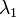$~\lambda_1$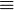$~\equiv$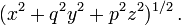$~(x^2 + q^2 y^2 + p^2 z^2)^{1 / 2} \, .$

When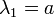$~\lambda_1 = a$, we obtain the standard definition of an ellipsoidal surface, it being understood that,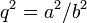$~q^2 = a^2/b^2$ and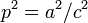$~p^2 = a^2/c^2$. (We will assume that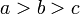$~a > b > c$, that is,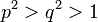$~p^2 > q^2 > 1$.) What is the expression for the unit vector normal to the surface at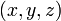$~(x, y, z)$ when written in terms of Cartesian unit vectors?

Well, to start with we know that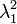$~\lambda_1^2$ is constant across the entire surface, so at any point on this specified surface we must find,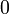$~0$$~=$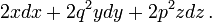$~2x dx + 2q^2y dy + 2p^2z dz \, .$